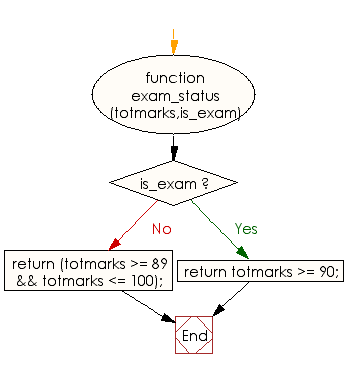# JavaScript: Check the total marks of a student in various examinations

## JavaScript Basic: Exercise-38 with Solution

Write a JavaScript program to check the total marks of a student in various examinations. The student will get A+ grade if the total marks are in the range 89..100 inclusive, if the examination is "Final-exam." the student will get A+ grade where total marks must be greater than or equal to 90. Return true if the student get A+ grade or false otherwise.

Sample Solution:

HTML Code:

``````<!DOCTYPE html>
<html>
<meta charset="utf-8">
<meta name="viewport" content="width=device-width">
<title>JavaScript program to check the total marks of a student in various examinations</title>
<body>

</body>
</html>
```
```

JavaScript Code:

``````function exam_status(totmarks,is_exam)
{
if (is_exam) {
}
return (totmarks >= 89 && totmarks <= 100);
}

console.log(exam_status("78", " "));
console.log(exam_status("89", "true "));
console.log(exam_status("99", "true "));
```
```

Sample Output:

```false
false
true
```

Flowchart:ES6 Version:

``````function exam_status(totmarks,is_exam)
{
if (is_exam) {
}
return (totmarks >= 89 && totmarks <= 100);
}

console.log(exam_status("78", " "));
console.log(exam_status("89", "true "));
console.log(exam_status("99", "true "));
``````

Live Demo:

See the Pen JavaScript: total marks of a student - basic-ex-38 by w3resource (@w3resource) on CodePen.

Improve this sample solution and post your code through Disqus

What is the difficulty level of this exercise?

Test your Programming skills with w3resource's quiz.

﻿

## JavaScript: Tips of the Day

Checks if a string is an anagram of another string (case-insensitive, ignores spaces, punctuation and special characters)

Example:

```const isAnagram = (str1, str2) => {
const normalize = str =>
str
.toLowerCase()
.replace(/[^a-z0-9]/gi, '')
.split('')
.sort()
.join('');
return normalize(str1) === normalize(str2);
};
console.log(isAnagram('iceman', 'cinema')); // true
```

Output:

```true
```